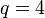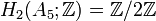# Group cohomology of alternating group:A5

This article gives specific information, namely, group cohomology, about a particular group, namely: alternating group:A5.
View group cohomology of particular groups | View other specific information about alternating group:A5

## Family contexts

Family name Parameter values General discussion of group cohomology of family
alternating group 5 group cohomology of alternating groups
projective general linear group of degree two over a finite field of size$q$$q = 4$, i.e., field:F4, so the group is$PGL(2,4)$ group cohomology of projective general linear group of degree two over a finite field
projective special linear group of degree two over a finite field of size$q$$q = 5$, i.e., field:F5, so the group is$PSL(2,5)$ group cohomology of projective special linear group of degree two over a finite field

## GAP implementation

### Computation of homology groups

The homology groups for trivial group action on the integers can be computed using the Hap package (if the package is installed but not automatically loaded, load it using LoadPackage("hap");), specifically its GroupHomology function. The function returns a list of numbers which are the orders of cyclic groups whose external direct product is the desired homology group.

#### First homology group

The first homology group, which is also the abelianization, can be computed as follows:

```gap> GroupHomology(AlternatingGroup(5),1);
[  ]```

This says that$H_1(A_5;\mathbb{Z}) = 0$.

#### Second homology group

The second homology group, which is also the Schur multiplier, can be computed as follows:

```gap> GroupHomology(AlternatingGroup(5),2);
[ 2 ]```

This says that$H_2(A_5;\mathbb{Z}) = \mathbb{Z}/2\mathbb{Z}$.

#### First few homology groups

```gap> List([1..12],i -> [i,GroupHomology(AlternatingGroup(5),i)]);
[ [ 1, [  ] ], [ 2, [ 2 ] ], [ 3, [ 2, 3, 5 ] ], [ 4, [  ] ], [ 5, [ 2, 2 ]
[ 6, [ 2 ] ], [ 7, [ 2, 3, 5 ] ], [ 8, [ 2, 2 ] ], [ 9, [ 2, 2 ] ],
[ 10, [ 2 ] ], [ 11, [ 2, 2, 2, 3, 5 ] ], [ 12, [ 2, 2 ] ] ]```